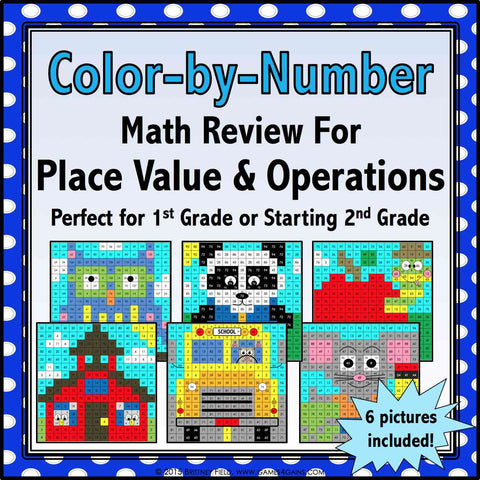## Back to School Color by Number - 1st/2nd Grade

• \$480

This back to school set comes with 6 "School Days" math color-by-number activities for reviewing and practicing place value and operations standards from 1st grade. This set is perfect to use as review with incoming 2nd graders or as practice with 1st graders who have already learned these skills.

Included with this "School Days" color-by-number set:
Instructions
6 color by number worksheets
6 student problem worksheets

Here are the Common Core standards covered by the color-by-number activities:

CCSS.MATH.CONTENT.1.NBT.A.1
Count to 120, starting at any number less than 120.

CCSS.MATH.CONTENT.1.NBT.B.2
Understand that the two digits of a two-digit number represent amounts of tens and ones.

CCSS.MATH.CONTENT.1.NBT.C.4
Add within 100, including adding a two-digit number and a one-digit number, and adding a two-digit number and a multiple of 10.

CCSS.MATH.CONTENT.1.NBT.C.5
Given a two-digit number, mentally find 10 more or 10 less than the number.

CCSS.MATH.CONTENT.1.NBT.C.6
Subtract multiples of 10 in the range 10-90 from multiples of 10 in the range 10-90.